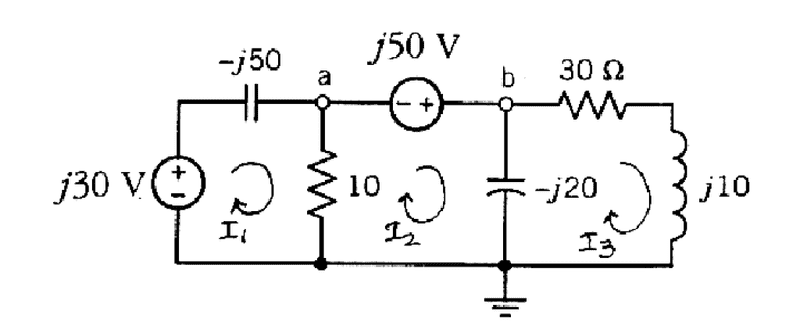Mesh Analysis

Homework StatementUse Mesh analysis to find voltage of Node A and B

V=IR
KVL

The Attempt at a Solution

Are my mesh equations correct?

Mesh1:
-j30V + (-j50 + 10)I1 - 10I2 = 0

Mesh2:
(10-j20)I2 - 10I1 - j50V - I3(-j20) = 0

Mesh3:
(-j20+30+j10)I3 - (-j20)I2 = 0

Last edited:

gneill
Mentor
Yes, your mesh current equations look fine.

Yes, your mesh current equations look fine.
How would I get the voltage of Node A and B?

gneill
Mentor
How would I get the voltage of Node A and B?
Ohm's law. You've solved for the currents so now you can find the potentials across any components you wish. Choose a path from the reference node to the location where you wish to know the potential and sum up the PD's along the way.

Ohm's law. You've solved for the currents so now you can find the potentials across any components you wish. Choose a path from the reference node to the location where you wish to know the potential and sum up the PD's along the way.
So if I wanted the voltage at Node A, I would multiple (I1-I2) with 10ohm resistor?

gneill
Mentor
So if I wanted the voltage at Node A, I would multiple (I1-I2) with 10ohm resistor?
That would work, yes. Any path from the reference node to a would work, and that happens to be a pretty convenient choiceThat would work, yes. Any path from the reference node to a would work, and that happens to be a pretty convenient choiceI ended up getting:
14.329 < -71.74 degrees for Va
Is that right?

Would Vb be: (I2-I3)*(-j20) -> 4.48 + 36.39j?

Last edited:
gneill
Mentor
I ended up getting:
14.329 < -71.74 degrees for Va
Is that right?

Would Vb be: (I2-I3)*(-j20) -> 4.48 + 36.39j?
They look good to me.

Note that for Vb you could also have just added j50 to Va; there is a fixed source of that amount tying them together.

•eehelp150